# NCERT Solutions For Class 11 Physics System of Particles and Rotational Motion Part-1## myCBSEguide App

CBSE, NCERT, JEE Main, NEET-UG, NDA, Exam Papers, Question Bank, NCERT Solutions, Exemplars, Revision Notes, Free Videos, MCQ Tests & more.

NCERT Solutions For Class 11 Physics System of Particles and Rotational Motion Part-1 Class 11 Physics Class book solutions are available in PDF format for free download. These ncert book chapter wise questions and answers are very helpful for CBSE exam. CBSE recommends NCERT books and most of the questions in CBSE exam are asked from NCERT text books. Class 11 Physics chapter wise NCERT solution for Physics part 1 and Physics part 2 for all the chapters can be downloaded from our website and myCBSEguide mobile app for free.

Download NCERT solutions for System of Particles and Rotational Motion Part-1  as PDF.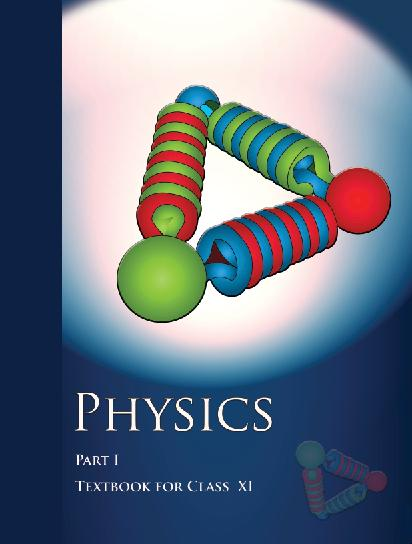## NCERT Class 11 Physics Chapter wise Solutions

• 1 – Physical World
• 2 – Units and Measurements
• 3 – Motion in a Straight line
• 4 – Motion in a Plane
• 5 – Laws of Motion
• 6 – Work, Energy and Power
• 7 – System of Particles and Rotational motion
• 8 – Gravitation
• 9 – Mechanical Properties of Solids
• 10 – Mechanical properties of fluids
• 11 – Thermal Properties of matter
• 12 – Thermodynamics
• 13 – Kinetic Theory
• 14 – Oscillations
• 15 – Waves

## CHAPTER SEVEN SYSTEM OF PARTICLES AND ROTATIONAL MOTION

• 7.1 Introduction
• 7.2 Centre of mass
• 7.3 Motion of centre of mass
• 7.4 Linear momentum of a system of particles
• 7.5 Vector product of two vectors
• 7.6 Angular velocity and its relation with linear velocity
• 7.7 Torque and angular momentum
• 7.8 Equilibrium of a rigid body
• 7.9 Moment of inertia
• 7.10 Theorems of perpendicular and parallel axes
• 7.11 Kinematics of rotational motion about a fixed axis
• 7.12 Dynamics of rotational motion about a fixed axis
• 7.13 Angular momentum in case of rotations about a fixed axis
• 7.14 Rolling motion

## NCERT Solutions For Class 11 Physics System of Particles and Rotational Motion Part-1

1. Give the location of the centre of mass of a (i) sphere, (ii) cylinder, (iii) ring, and (iv) cube, each of uniform mass density. Does the centre of mass of a body necessarily lie inside the body?

2. In the HCl molecule, the separation between the nuclei of the two atoms is about 1.27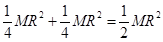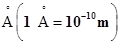. Find the approximate location of the CM of the molecule, given that a chlorine atom is about 35.5 times as massive as a hydrogen atom and nearly all the mass of an atom is concentrated in its nucleus.

3. A child sits stationary at one end of a long trolley moving uniformly with a speed V on a smooth horizontal floor. If the child gets up and runs about on the trolley in any manner, what is the speed of the CM of the (trolley + child) system?

4. Show that the area of the triangle contained between the vectors a and b is one half of the magnitude of a x b.

5. Show that a.(b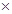c) is equal in magnitude to the volume of the parallelepiped formed on the three vectors, a, b and c.

6. Find the components along the x, y, z axes of the angular momentum l of a particle, whose position vector is r with components x, y, z and momentum is p with components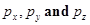. Show that if the particle moves only in the xy plane the angular momentum has only a z-component.

7. Two particles, each of mass m and speed v, travel in opposite directions along parallel lines separated by a distance d. Show that the vector angular momentum of the two particle system is the same whatever be the point about which the angular momentum is taken.

8. A non-uniform bar of weight W is suspended at rest by two strings of negligible weight as shown in Fig.7.39. The angles made by the strings with the vertical are 36.9° and 53.1° respectively. The bar is 2 m long. Calculate the distance d of the centre of gravity of the bar from its left end.

9. A car weighs 1800 kg. The distance between its front and back axles is 1.8 m. Its centre of gravity is 1.05 m behind the front axle. Determine the force exerted by the level ground on each front wheel and each back wheel.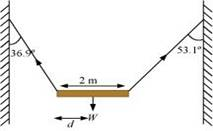10. (a) Find the moment of inertia of a sphere about a tangent to the sphere, given the moment of inertia of the sphere about any of its diameters to be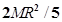, where M is the mass of the sphere and R is the radius of the sphere.

(b) Given the moment of inertia of a disc of mass M and radius R about any of its diameters to be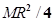, find its moment of inertia about an axis normal to the disc and passing through a point on its edge.

11. Torques of equal magnitude is applied to a hollow cylinder and a solid sphere, both having the same mass and radius. The cylinder is free to rotate about its standard axis of symmetry, and the sphere is free to rotate about an axis passing through its centre. Which of the two will acquire a greater angular speed after a given time?

12. A solid cylinder of mass 20 kg rotates about its axis with angular speed 100 rad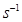. The radius of the cylinder is 0.25 m. What is the kinetic energy associated with the rotation of the cylinder? What is the magnitude of angular momentum of the cylinder about its axis?

13. (a) A child stands at the centre of a turntable with his two arms outstretched. The turntable is set rotating with an angular speed of 40 rev/min. How much is the angular speed of the child if he folds his hands back and thereby reduces his moment of inertia to 2/5 times the initial value? Assume that the turntable rotates without friction.

(b) Show that the child’s new kinetic energy of rotation is more than the initial kinetic energy of rotation. How do you account for this increase in kinetic energy?

14. A rope of negligible mass is wound round a hollow cylinder of mass 3 kg and radius 40 cm. What is the angular acceleration of the cylinder if the rope is pulled with a force of 30 N? What is the linear acceleration of the rope? Assume that there is no slipping.

15. To maintain a rotor at a uniform angular speed of 200 rad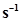, an engine needs to transmit a torque of 180 Nm. What is the power required by the engine?

(Note: uniform angular velocity in the absence of friction implies zero torque. In practice, applied torque is needed to counter frictional torque). Assume that the engine is 100 % efficient.

16. From a uniform disk of radius R, a circular hole of radius R/2 is cut out. The centre of the hole is at R/2 from the centre of the original disc. Locate the centre of gravity of the resulting flat body.

17. A metre stick is balanced on a knife edge at its centre. When two coins, each of mass 5 g are put one on top of the other at the 12.0 cm mark, the stick is found to be balanced at 45.0 cm. What is the mass of the metre stick?

18. A solid sphere rolls down two different inclined planes of the same heights but different angles of inclination. (a) Will it reach the bottom with the same speed in each case? (b) Will it take longer to roll down one plane than the other? (c) If so, which one and why?

19. A hoop of radius 2 m weighs 100 kg. It rolls along a horizontal floor so that its centre of mass has a speed of 20 cm/s. How much work has to be done to stop it?

20. The oxygen molecule has a mass of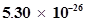kg and a moment of inertia of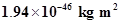about an axis through its centre perpendicular to the lines joining the two atoms. Suppose the mean speed of such a molecule in a gas is 500 m/s and that its kinetic energy of rotation is two thirds of its kinetic energy of translation. Find the average angular velocity of the molecule.

21. A solid cylinder rolls up an inclined plane of angle of inclination 30°. At the bottom of the inclined plane the centre of mass of the cylinder has a speed of 5 m/s.

(a) How far will the cylinder go up the plane?

(b) How long will it take to return to the bottom?

## NCERT Solutions for Class 11 Physics

NCERT Solutions Class 11 Physics PDF (Download) Free from myCBSEguide app and myCBSEguide website. Ncert solution class 11 physics includes text book solutions from both part 1 and part 2. NCERT Solutions for CBSE Class 11 Physics have total 15 chapters. 11 Physics NCERT Solutions in PDF for free Download on our website. Ncert physics class 11 solutions PDF and physics ncert class 11 PDF solutions with latest modifications and as per the latest CBSE syllabus are only available in myCBSEguide

## CBSE app for Class 11

To download NCERT Solutions for class 11 Physics, Chemistry, Biology, History, Political Science, Economics, Geography, Computer Science, Home Science, Accountancy, Business Studies and Home Science; do check myCBSEguide app or website. myCBSEguide provides sample papers with solution, test papers for chapter-wise practice, NCERT solutions, NCERT Exemplar solutions, quick revision notes for ready reference, CBSE guess papers and CBSE important question papers. Sample Paper all are made available through the best app for CBSE students and myCBSEguide website